Courses

# Types of Force Class 9 Notes | EduRev

## Science Class 9

Created by: Dr Manju Sen

## Class 9 : Types of Force Class 9 Notes | EduRev

The document Types of Force Class 9 Notes | EduRev is a part of the Class 9 Course Science Class 9.
All you need of Class 9 at this link: Class 9

Force: From our day to day experience, we all are familiar with the concept of 'Force'. While opening a door, lifting a bucket or a bag, kicking a football or throwing a stone, we have to act differently and in many ways. We are either pushing or pulling the object. The no non-living object moves on its own. We have to apply the effort in the form of push or pull. This pull or push is called force. Thus a force is an external agency that displaces or tends to displace a body from its position of rest. The direction in which the object is pushed or pulled is called the direction of the force. Force has both magnitude and direction. It is a vector quantity.

___________________

Force: Force is the 'push' or 'pull' which can make a body move, stop a moving body, change the direction and speed of a moving body. Force cannot be seen but the effect of force on an object can be seen or felt.

___________________

Definition of Force

A force is a physical quantity which causes or tends to cause motion in an object at rest or changes or tends to change the direction of motion of a moving object or changes or tends to change the size and shape of an object or change or tends to change the speed of an object.

Effect of force
A force produces the following effects:-

• It can move or tend to move an object.
• It can stop or tend to stop an object in motion.
• It can change or tend to change the direction of motion of an object.
• It can increase or decrease the speed of an object.
• It can change the shape or size of an object.

Force can move or tend to move an object
When a force is applied to a stationary body, it can either move the body or tend to move it. The motion of the body takes place in the direction of the applied force. Some examples of this are :
(i) When a football is kicked it is set into motion.
(ii) A railway locomotive applies force on the railway wagons to set them into motion.
(iii) A horse applies force on the cart to pull it.
(iv) At times when you push a wall, you are unable to move it. The force applied by use tends to move the wall.

Force can stop or tend to stop an object
When a force is applied on a moving body, it can either stop the body or tend to stop it. For this a force is applied opposite to the direction of motion of the body. Some examples of this are :
(i) When a football in motion has to be stopped a force is applied opposite to the direction of motion of the football.
(ii) A railway locomotive applies brakes on the railway wagons to stop them.
(iii) A rolling body comes to rest due to frictional force. This force acts opposite to the direction of motion of the body.
(iv) At times when you push a car in motion, you are unable to stop it. The force applied by use tends to stop the moving car.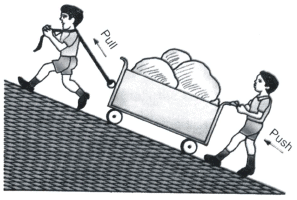Force can change or tend to change the direction of motion of an object
(i) When a football in motion kicked perpendicular to its motion it's direction of motion changes.
(ii) A perpendicular force is required to make a particle move in a circular path. This force is called centripetal force. This force continuously changes the direction of motion of the body.
(iii) A cricketer applies a tangential force to change the direction of the cricket ball.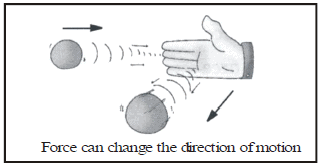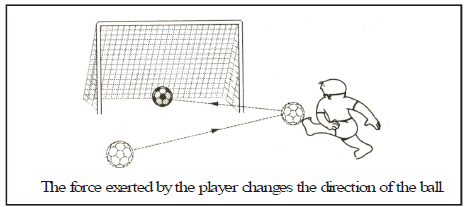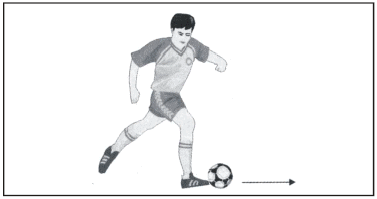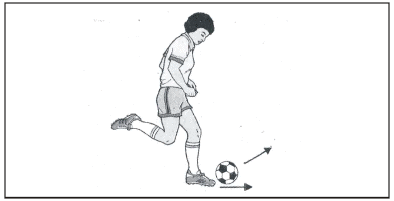Force can increase or decrease the speed of an object

When a force is applied on a moving body, in the direction of motion or opposite to it, it can change the speed of the object. Some examples of this are :
(i) When a football in motion is kicked in the direction of its motion its speed increases.
(ii) The speed of a car can be decreased by applying brakes.
(iii) The speed of a cycle can be increased by applying force on the pedals.
(iv) A freely falling object is accelerated towards the earth due to the gravitational attraction of the earth.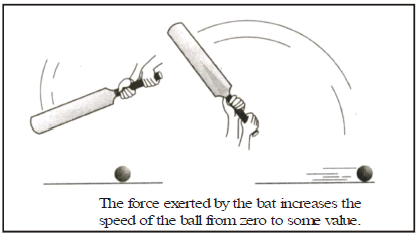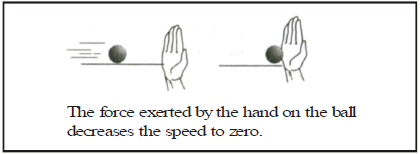Effect on Shape

Ex.1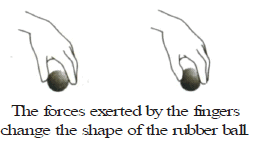Ex.2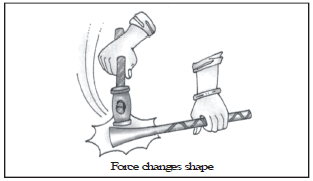Ex.3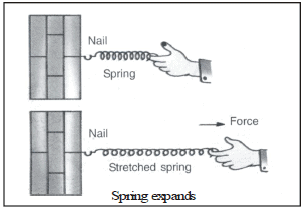The concept of force
The concept of force gives us the quantitative description of the interaction between two bodies or between a body and the environment.
When you push or pull a car stuck in mud, you exert a force on it. This force may or may not be able to move the car.
Thus force is an agent (often termed as a pull or a push), which

• Can move or tend to move an object.
• Can stop or tend to stop an object in motion.
• Can change or tend to change the direction of motion of an object.
• Can increase or decrease the speed of an object.
• From all these cases we can conclude that force is actually producing acceleration.

Thus, force is an agent whose action can produce acceleration in a body.
A force can also change the shape of an object on which it is acting. For example when you squeeze a soft rubber ball it will be deformed to some extent.

In other words a force can be defined as an agent which, can change or tend to change the dimension of an object or produces or tends to produce acceleration in an object.
Broadly speaking forces are classified as being of two types. (i) Contact force, (ii) Non-contact force

Contact force: The force which acts on a body either directly or through some connector are called contact forces.
Examples: Biological force, muscular force, mechanical force and frictional force are the examples of contact force.

MUSCULAR FORCE
This force produced by the muscles of living beings is called muscular force or biological force.

Mechanical Force
The force generated by machines is called mechanical force.

FRICTION FORCE :
A force which acts at the surface of contact, when one body moves or tends to move upon another body is called the force of friction.
The force of friction has the following properties :
(i) It is produced only when one body is made to move upon another body.
(ii) It always opposes the motion of the body and tries to stop the body.
(iii) It wears off the surface of contact of two bodies.
It is the force of friction which makes it difficult for us to move heavy objects such as large boxes; carts laden with different kinds of substances, etc. Again, it is the force of friction which wears off the tires of our bicycles, cars, trucks, etc.

Non-contact Force or Field force :
The force which does not make direct contact with a body and acts through space, without any connector is called non-contact force.

Examples:
(i) The force of gravity of the earth is a non-contact force which attracts all bodies towards the earth.
(ii) The electrostatics force can pull tiny bits of dry paper is a non-contact force.
(iii) A magnet can attract common pins of steel from a distance and hence the magnetic force is non-contact force.

Gravitational Force:-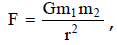Here G is a universal gravitational constant & m1 m2 are masses, and r is a distance between two bodies.

Electrostatics Force:-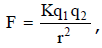Here K is a Coulomb's constant & q1 q2 are charges, and r is a distance between two charges.

MAGNETIC FORCE: The strange property of some substances to attract iron or steel objects towards itself is called magnetic property. The substance which has the above property is called a magnet.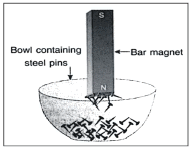As the objects of iron or steel move towards the magnet, therefore we can say that they are acted upon by the force of the magnet.
Resultant force: If a single force acting on a body produces the same acceleration as produced by a number of forces, that single force is called the resultant of these individual force.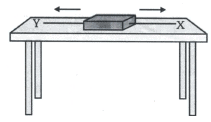Normal Force:-
The force acting perpendicular to the ground surface then the force is called normal force.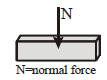Balanced and unbalanced forces:
If a set of forces acting on a body produces no acceleration in it, the forces are said to be balanced. If it produces a nonzero acceleration, the forces are said to be unbalanced.

Definition of balance force:-
When two forces of equal magnitude but acting in opposite directions on an object simultaneously then the object continues in its state of rest of uniform motion in a straight line. Such forces acting on the object are known as balance force.

Examples of balanced force:-
1. When we push a wall, the wall does not move at all i.e., it remains at rest.
In this case, the force of push on the wall acts in the forward direction. On the other hand, the wall also exerts equal force on our hand in the backward direction. These two equal and opposite forces cancel out the effect of each other and hence these two forces are the balanced forces.

2. When we try to push a heavy box on a rough surface, it does not move.

3. In a tug of war, two terms pull a rope in opposite directions. If the rope does not move in any direction in spite of the fact that both the teams are applying forces in opposite directions, then the forces applied by both the teams are known as balanced forces.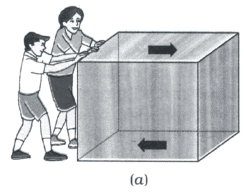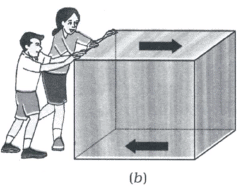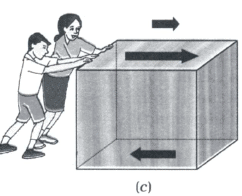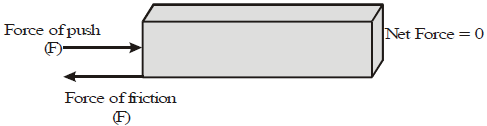Definition of Unbalanced force:-
When two forces of unequal magnitudes act in opposite directions on an object simultaneously then the object move in the direction of a large force. These forces acting on the object are known as unbalanced forces.

Examples of unbalanced force:-
1. When a boy drags a box on the floor, then an unbalanced force is acting on the box.
2. A bicycle will slow down if the rider stops pedaling it.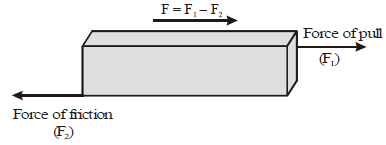## Science Class 9

205 videos|285 docs|135 tests

,

,

,

,

,

,

,

,

,

,

,

,

,

,

,

,

,

,

,

,

,

;